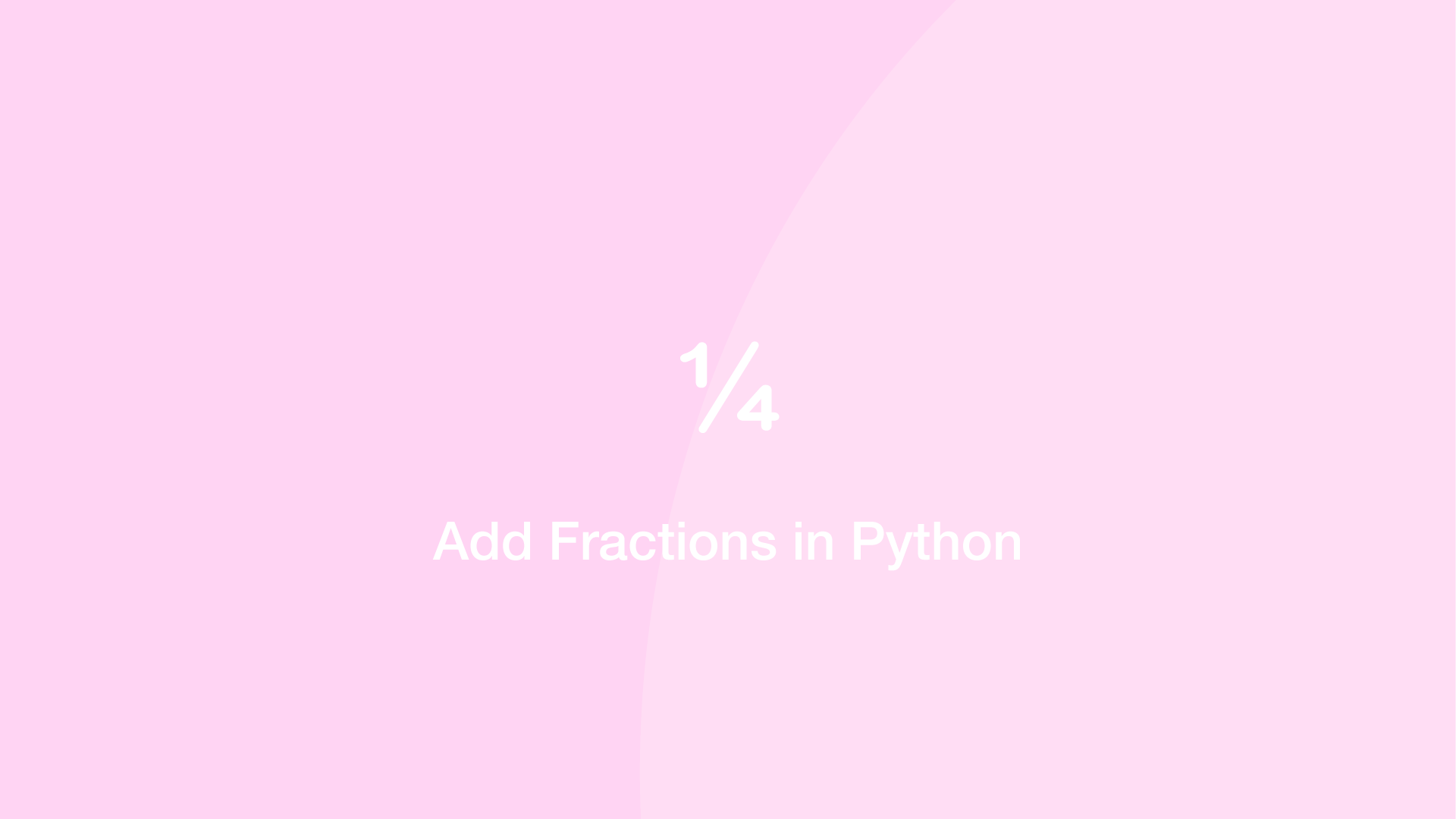How to Add Fractions in PythonTo add fractions in Python, import the Fractions module, pass each fraction component to the Fraction function as arguments then add the fraction objects together.

Here is an example of adding 1/2 and 1/4 together:

from fractions import Fraction

result = Fraction(1,2) + Fraction(1,4)

print(result)
3/4

You don't have to supply the fraction components as arguments. You could just supply formatted fraction strings and the Fractions function will do the leg work:

from fractions import Fraction

result = Fraction('1/2') + Fraction('1/4')

print(result)
3/4

Related TutorialsHow to use Sets in Python

September 15, 2020How to Use Argparse in Python

September 25, 2020How to Create and Use Lists (Arrays) in Python

September 01, 2020How to Change the Default Python Version to Python 3.9

December 17, 2020How to Use While Loops in Python

September 03, 2020October 13, 2020